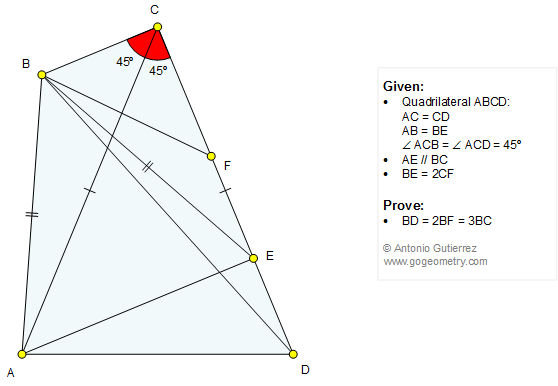# Geometry Problem 1324: Quadrilateral, Diagonal, 45 Degrees, Angle Bisector, Isosceles Triangle, Congruence. Level: High School, Honors Geometry, College, Mathematics Education

< PREVIOUS PROBLEM  |  NEXT PROBLEM >

 The figure shows a quadrilateral ABCD with AC = CD and angle ACB = angle ACD = 45 degrees. Points F and E are on CD so that AE is parallel to BC, AB = BE and BE = 2CF. Prove that BD = 2BF = 3BC.This entry contributed by Sumith Peiris, Moratuwa, Sri Lanka.See also: Art and typography of problem 1324
 Home | Search | Geometry | Problems | All Problems | Open Problems | Visual Index | Problems Art Gallery | Ten problems: 1321-1330 | Triangles | Isosceles triangle | Quadrilaterals | 45 degrees | Angle Bisector | Angles | Email | Post a comment or solution